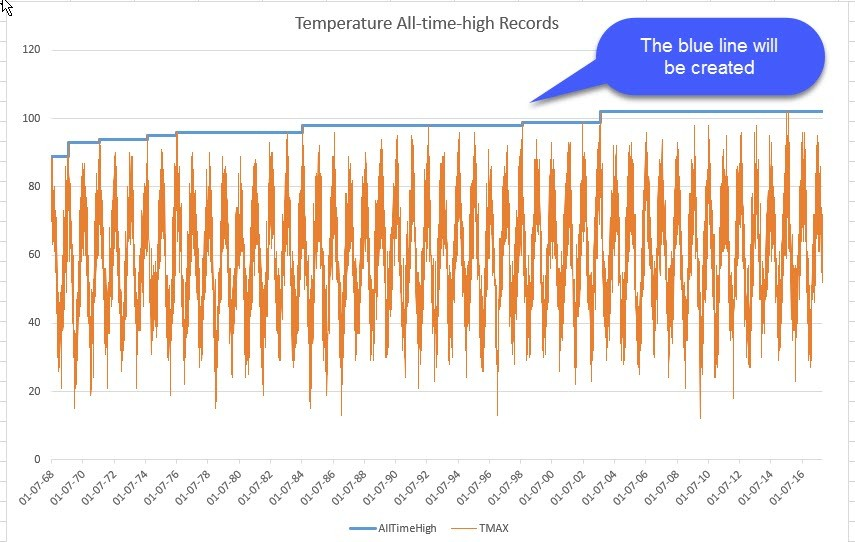# Table.Group: Exploring the 5th element in Power BI and Power QueryIn this post I’ll show you the magic stuff you can do with the 5th parameter (the optional comparer function) of the Table.Group M-function in Power BI and Power Query:

### Table.Group parameters

1. table as table,
2. key as any,
3. aggregatedColumns as list,
4. optional groupKind as nullable number,
5. optional comparer as nullable function

If you’re not familiar with the 4th parameter (groupKind) already, I strongly recommend to read Chris Webb’s article, as we will build on its knowledge here.

Another aspect worth mentioning for the modus in GroupKind.local is the performance aspect: It runs MUCH faster for large datasets than the default-setting. So if you are sure that your data will always be sorted accordingly, you can speed up your grouping-operations considerably. That means: Your data has to be sorted correctly by default. At least for my tests, you would loose the performance-gain once you’d sort your table by an explicit step before.

You can find an overview of comparer functions here.

### Case insensitive grouping

Imagine there was a twist in Chris’ dataset and it would look like so:

We would probably not be happy with these results then:

Because M is by default case sensitive, we get more groups than we want. Let’s try Comparer.OrdinalIgnoreCase to the rescue then:Pretty neat, isn’t it 😉  (You can use that comparer in other functions as well, see for text- and list operations)

Something like this was what I’ve showed Huang Caiguang the other day, who asked me what the 5th parameter of this function was about (or so I understood). He then sent me a link to one of his articles, which demanded a good 2 hours for me to digest and understand: We can also use custom functions to create all different sorts of grouping behaviours here. These are my 2 favourites:

#### 1. Analysing all-time-records#### 2. Analyzing events between

Let’s adjust the dataset again a bit and add some more vacations:

Say we want to analyse everything that happend between each vacation:

### But how does this work?

I don’t know exactly, but here is my guess:

The Table.Group-function will pass 2 parameters to the function in the 5th arguments if it is used: For GroupKind.Local this is group-columns-record from the initial/first row of the table/group and the respective record of the current row.

As long as the comparer-function returns 0, the current row will be regarded as belonging to the group: This is a match in the Comparer.OrdinalIgnoreCase-function and also the value of false (which makes the syntax a bit counterintuitive here in my eyes)

#### 1. For the All-time-record:

`Table.Group(#"Changed Type", {"TMAX"}, <Aggregated columns expressions>, 0, (x,y) => Number.From(x[TMAX]<y[TMAX]))`

We check if the temperature of the current record is higher than the initial record of the current group. If true, the result will be 1 and a new group will be created.

#### 2. For the events between:

`Table.Group(FilteredRows, "Activity", <Aggregated columns expressions>, 0, (x,y) => Number.From(x=y))`

Here we check the equality of the full records and as long as they are NOT equal, 0 will be returned and they will stay in the group. Just when the same value than the first record of the group (“Vacation”) is returned, a new group will be created. I haven’t found out how to include the Comparer.OrdinalIgnoreCase into this syntax, but if you want to make this case-insensitive, you can transform the arguments to lowercase like this: … (x,y) => Number.From(Text.Lower(x) = Text.Lower(y)) (provided that the key (2nd parameter) is a text and not a list (“Activity” instead of {“Activity”}).

If you check query “Total Activity Explore” in the sample file, you will notice that the arguments that will be passed into the 5th parameter for the global group kind are pretty different: More fields in the record and different row-order.

Do you have other use cases to create groups based on a consecutive order which compares items with the first item of each group? Please share them in the comments.

Enjoy & stay queryious 😉

### Comments (10) Write a comment

1.It would be really useful if we can get to work in 6th parameter of Table.Join

2.Hello
I would prefer = Table.Group(#”Changed Type”, {“TMAX”}, {{“All”, each _, type table}}, 0, (current,group) => if Value.Compare(current[TMAX],group[TMAX])= -1 then 1 else 0)
Table.Group(#”Changed Type”, {“TMAX”}, , 0, (x,y) => Number.From(x[TMAX]<y[TMAX]))
and use a if then else statement to check. This is a way more readable… so fi current < group (-1 of value compare use 1 (for including in group) and 0 for not including
Jimmy

3.here:

https://community.powerbi.com/t5/Power-Query/Dynamic-data-transformation-with-complex-pivoted-dataset/m-p/1196623

a solution using the fifth element of table.group may be valid:

Table.Group(#”Replaced Value”, {“Location”}, {{“Count”, each Table.RowCount(), type number}, {“blk”, each Table.AddColumn(,”supLocation”, (r)=> if not List.Contains({“Total”,”Other”,”#”},r[Location]) then _[Location]{1} else r[Location]) }},GroupKind.Local,(x,y)=>Number.From(x=y))

•Great to see that you’re using my technique to help others !!

4.I used this technique here

https://community.powerbi.com/t5/Power-Query/Combine-multiple-rows-in-one-in-bank-statement-just-for-one/td-p/1201411

to solve the proposed problem.

this is the expression that has been accepted as a solution:

Table.Group(name_tab_previuo_step, {“Date”}, {{“nop”, each Text.Combine([#”Nature de l’opération”],” “)},{“Debito”, each List.First([Débit])}},GroupKind.Local,(x,y)=>Number.From((x=y or y[Date]<>null)))

•Well done!

5.